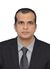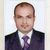# QlikView App Dev

Discussion Board for collaboration related to QlikView App Development.

Announcements
QlikWorld 2023, a live, in-person thrill ride. Save \$300 before February 6: REGISTER NOW!
cancel
Showing results for
Did you mean:Not applicable

## Regarding two date fields in a master calendar

Hello Friends,

Kindly let me know your valuable suggestions. I have a master calendar created with a date field called PR_Date which is linked to fact table using PR_Date.

Min_Max:
Min([_Date - PR])         as MinDate,
Max([_Date - PR])         as MaxDate

LET vMinDate = Peek('MinDate', 0, 'Min_Max');
LET vMaxDate = Peek('MaxDate', 0, 'Min_Max');

DROP TABLE Min_Max;

[CalendarPR]:
Dual(YearName(Temp_Date,0,\$(vFM)), year(YearStart(Temp_Date,0,\$(vFM))))
as [Year PR],
as [fYear PR],
YearName(Temp_Date,0,\$(vFM))
as [fYearName PR],
Year(Temp_Date)           as [CalendarYear PR],
Dual('Q' & ceil((Mod(Month(Temp_Date)-\$(vFM), 12)+1)/3), ceil((Mod(Month(Temp_Date)-\$(vFM), 12)+1)/3))
as [Qtr PR],
Date(WeekStart(Temp_Date),'YYYY-MMM-DD')
as [RWeek PR],
Date(WeekEnd(Temp_Date),'YYYY-MMM-DD')
as [RWeekEnd PR],
Div((Date(WeekStart(Temp_Date),'YYYY-MMM-DD')-Date(WeekStart(YearStart(Temp_Date,0,\$(vFM))),'YYYY-MMM-DD') + 7),7)
as [WeekNo PR],
Week(Temp_Date)           as [CalendarWeekNo PR],
Dual(Month(Temp_Date), Mod(Month(Temp_Date)-\$(vFM), 12)+1)
as [Month PR],
Mod(Month(Temp_Date)-\$(vFM), 12)+1
as [fMonth PR],
Month(Temp_Date)          as [CalendarMonth PR],
Date(MonthStart(Temp_Date),'YYYY-MMM')
as [RMonth PR],
Day(Temp_Date)            as [Day PR],
Date(Temp_Date)           as [Date - PR],
Temp_Date                 as [_Date - PR]
;
(
\$(vMinDate) + IterNo() - 1)
as Temp_Date
AUTOGENERATE (1)
WHILE \$(vMinDate) + IterNo() - 1 <= RangeMin(\$(vMaxDate),AddYears(Today(1),2));

LET vMinDate = Null();
LET vMaxDate = Null();
Let vFM=null();

Now they want to include another date field called PO_Date , so I created the same calendar with PO_Date in another tab and linked to fact table using PO_Date as follows

Min_Max:
Min([_Date - PO])         as MinDate,
Max([_Date - PO])         as MaxDate

LET vMinDate = Peek('MinDate', 0, 'Min_Max');
LET vMaxDate = Peek('MaxDate', 0, 'Min_Max');

DROP TABLE Min_Max;
SET vFM = 4;
[CalendarPO]:
Dual(YearName(Temp_Date,0,\$(vFM)), year(YearStart(Temp_Date,0,\$(vFM))))
as [Year PO],
as [fYear PO],
YearName(Temp_Date,0,\$(vFM))
as [fYearName PO],
Year(Temp_Date)           as [CalendarYear PO],
Dual('Q' & ceil((Mod(Month(Temp_Date)-\$(vFM), 12)+1)/3), ceil((Mod(Month(Temp_Date)-\$(vFM), 12)+1)/3))
as [Qtr PO],
Date(WeekStart(Temp_Date),'YYYY-MMM-DD')
as [RWeek PO],
Date(WeekEnd(Temp_Date),'YYYY-MMM-DD')
as [RWeekEnd PO],
Div((Date(WeekStart(Temp_Date),'YYYY-MMM-DD')-Date(WeekStart(YearStart(Temp_Date,0,\$(vFM))),'YYYY-MMM-DD') + 7),7)
as [WeekNo PO],
Week(Temp_Date)           as [CalendarWeekNo PO],
Dual(Month(Temp_Date), Mod(Month(Temp_Date)-\$(vFM), 12)+1)
as [Month PO],
Mod(Month(Temp_Date)-\$(vFM), 12)+1
as [fMonth PO],
Month(Temp_Date)          as [CalendarMonth PO],
Date(MonthStart(Temp_Date),'YYYY-MMM')
as [RMonth PO],
Day(Temp_Date)            as [Day PO],
Date(Temp_Date)           as [Date - PO],
Temp_Date                 as [_Date - PO]
;
(
\$(vMinDate) + IterNo() - 1)
as Temp_Date
AUTOGENERATE (1)
WHILE \$(vMinDate) + IterNo() - 1 <= RangeMin(\$(vMaxDate),AddYears(Today(1),2));

LET vMinDate = Null();
LET vMaxDate = Null();
Let vFM=null();

It is working absolutely fine, but they want me to concatenate both as a single table instead of two. When I insert a concatenate between the two tables and reload the above code is giving an error.

Kindly let me know your valuable suggestions to make the above two table calendars into one table calendar based on both the date fields PR_Date and PO_Date.

Thanks so much and I sincerely appreciate your time.

Best regards,

Kiru

5 RepliesMVPNot applicable
Author

Hello Manish,

My apology for the delay.  Thanks for the information, but is it possible to concatenate all the different master calendars into one single calendar?

Kind regards,

KiruMVP

You need to use Canonical Date which is creating a single calendar for different dates and using Flag to identify the different tables.Champion III

Hi,

Check this too.

Regards

ASHFAQMVP

Hi,

As to see your script it needs a common date if you create a common date and create a master calender by using canonical dates.